## numerical

 Question 1

Two cars start at the same time from the same location and go in the same direction. The speed of the first car is 50 Km/h and the speed of the second car is 60 Km/h. The number of hours it takes for the distance between the two cars to be 20 Km is _____.

 A 2 B 3 C 1 D 6
Aptitude       Numerical       GATE 2019       Video-Explanation
Question 1 Explanation:
Speed of the first car = 50 km/h
Speed of the second car = 60 km/h
Let no. of hours = x say
⇒ From the question we can write
(60)x - (50)x = 20
10x = 20
∴ x = 2 hrs
 Question 2

In a college, there are three student clubs. Sixty students are only in the Drama club, 80 students are only in the Dance club, 30 students are only in the Maths club, 40 students are in both Drama and Dance clubs, 12 students are in both Dance and Maths clubs, 7 students are in both Drama and Maths club, and 2 students are in all the clubs. If 75% of the students in the college are not in any of these clubs, then the total number of students in the college is _____.

 A 900 B 975 C 225 D 1000
Aptitude       Numerical       GATE 2019       Video-Explanation
Question 2 Explanation:No. of students present in three student clubs
= 60 + 80 + 30 + 38 + 2 + 10 + 5
= 225 [i.e., 25% of the students in the college]
Total no. of students in the college = 225 × 4 = 900
 Question 3

Three of the five students allocated to a hostel put in special requests to the warden. Given the floor plan of the vacant rooms, select the allocation plan that will accommodate all their requests.

Request by X: Due to pollen allergy, I want to avoid a wing next to the garden.
Request by Y: I want to live as far from the washrooms as possible, since I am very sensitive to smell.
Request by Z: I believe in Vaastu and so want to stay in the South-west wing.

 A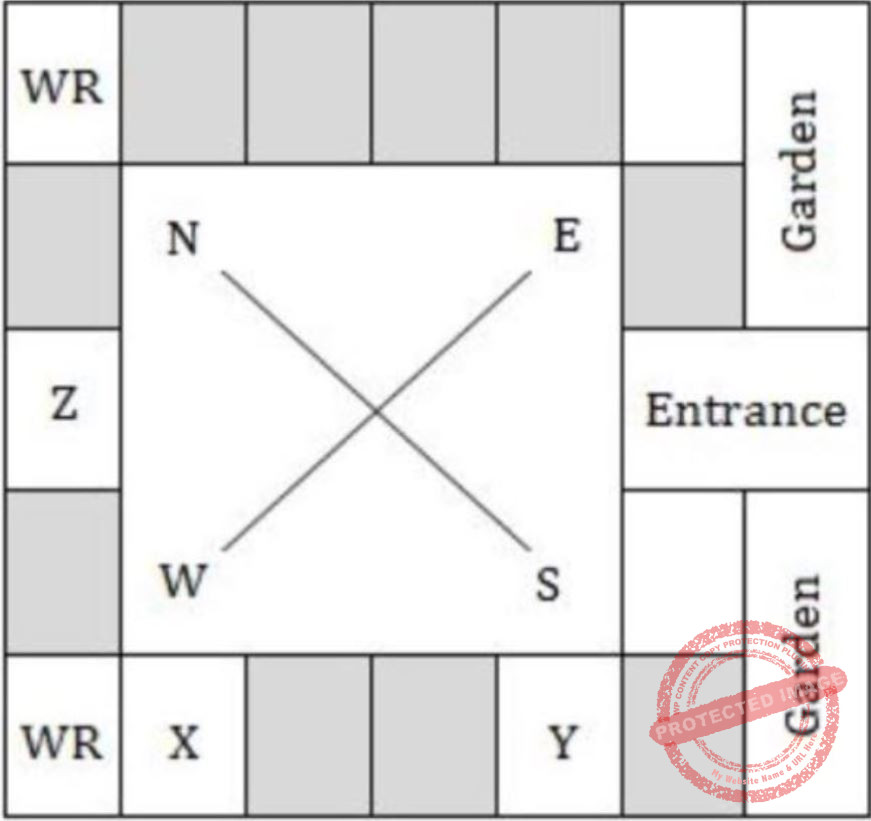BCDAptitude       Numerical       GATE 2019       Video-Explanation
Question 3 Explanation:
Consider Option D, which satisfies all the given three statements.
 Question 4

In the given diagram, teachers are represented in the triangle, researchers in the circle and administrators in the rectangle. Out of the total number of the people, the percentage of administrators shall be in the range of _____.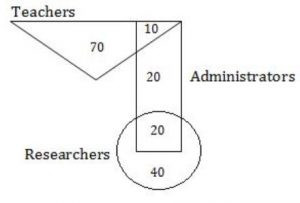A 16 to 30 B 0 to 15 C 46 to 60 D 31 to 45
Aptitude       Numerical       GATE 2019       Video-Explanation
Question 4 Explanation: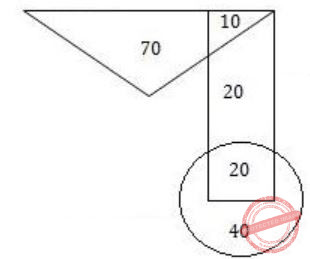From the given diagram:Total no. of people = 70 + 10 + 20 + 20 + 40 = 160
% of Administrators = 50/160 = 31.25
 Question 5

The area of a square is d. What is the area of the circle which has the diagonal of the square as its diameter?

 A πd B πd2 C (1/2)πd2 D (1/2)πd
Aptitude       Numerical       GATE 2018       Video-Explanation
Question 5 Explanation:
In general,
Area of a square A = a2 (where a is side)
In the question,
Area of a square = d
The side of a square = √d
Diagonal of a square = Diameter of circle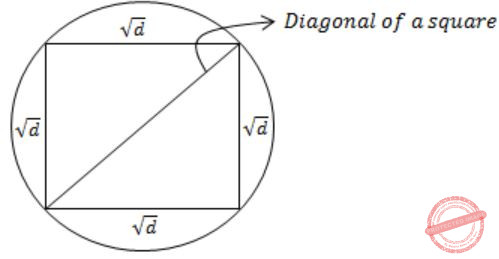From Pythagoras theorem,Question 6

What is the missing number in the following sequence?

2, 12, 60, 240, 720, 1440, _______, 0
 A 2880 B 1440 C 720 D 0
Aptitude       Numerical       GATE 2018       Video-Explanation
Question 6 Explanation:2 × 6 = 12
12 × 5 = 60
60 × 4 = 240
240 × 3 = 720
720 × 2 = 14401440 × 0 = 0
 Question 7

What would be the smallest natural number which when divided either by 20 or by 42 or by 76 leaves a remainder of '7' in each case?

 A 3047 B 6047 C 7987 D 63847
Aptitude       Numerical       GATE 2018       Video-Explanation
Question 7 Explanation:
Method-I:
Take the LCM of 20, 42, 76 i.e., 7980.
⟹ Remainder = 7
⟹ Smallest number divisible by 20 (or) 42 (or) 72 which leaves remainder 7 is = 7980 + 7 = 7987
Method-II:
Option I:
3047 – 7 = 3040
3040 not divisible by 42
Option II:
6040 not divisible by 42
Option III:
7980 divisible by 20, 42, 76
Option IV:
63840 divisible by 20, 42, 76
→ Smallest (7980, 63840) = 7980
→ 7980 + remainder 7 = 7987
 Question 8

If pqr ≠ 0 and p -x = 1/q, q -y = 1/r, r -z = 1/p, what is the value of the product xyz?

 A -1 B 1/pqr C 1 D pqr
Aptitude       Numerical       GATE 2018       Video-Explanation
Question 8 Explanation:
pqr≠0
→ p-x = 1/q ⟹ 1/px = 1/q
⟹ q = px
⟹ log q = log px
⟹ x log p = log q
⟹ x = log q/ log p
→ q-y = 1/r ⟹ 1/qy = 1/r
⟹ qy = r
⟹ y log q = log r
⟹ y = log r/ log q
→ r-z = 1/p ⟹ 1/rz = 1/p
⟹ p = rz
⟹ log rz = log p
⟹ z log r = log p
⟹ z = log p/ log r
XYZ = log q/ log p * log r/ log q * log p/ log r =1
 Question 9

In the figure below, ∠DEC + ∠BFC is equal to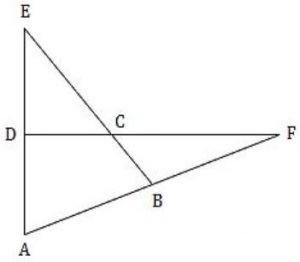Aptitude       Numerical       GATE 2018       Video-Explanation
Question 9 Explanation:→ ∠1 = ∠5 + ∠4 ………(i)
According to triangular property:
Angle of exterior = sum of interior angles
→ ∠4 = ∠3 + ∠2 ……….(ii)
By substituting (ii) in (i)
→ ∠1 = ∠5 + ∠3 + ∠2
→ ∠1 = ∠BCD
→ ∠BCD - ∠BAD = ∠1 - ∠2
= ∠5 + ∠3 + ∠2 - ∠2
= ∠ 5 + ∠3
= ∠DEC + ∠BFC
 Question 10

In a party, 60% of the invited guests are male and 40% are female. If 80% of the invited guests attended the party and if all the invited female guests attended, what would be the ratio of males to females among the attendees in the party?

 A 2:3 B 1:1 C 3:2 D 2:1
Aptitude       Numerical       GATE 2018       Video-Explanation
Question 10 Explanation:
Let us consider total no. of guests = 100
→ 60% invited guests are males i.e., 60 males
→ 40% invited guests are females i.e., 40 females
→ 80% invited guests are attended i.e., total guests attended to a party = 80
→ All the invited females are attended then remaining people are males = 80 – 40 = 40 males
⇒ 40 males : 40 females
⇒ 1 : 1
 Question 11

In appreciation of the social improvements completed in a town, a wealthy philanthropist decided to gift Rs 750 to each male senior citizen in the town and Rs 1000 to each female senior citizen. Altogether, there were 300 senior citizens eligible for this gift. However, only 8/9th of the eligible men and 2/3rd of the eligible women claimed the gift. How much money (in Rupees) did the philanthropist give away in total?

 A 1,50,000 B 2,00,000 C 1,75,000 D 1,51,000
Aptitude       Numerical       GATE 2018       Video-Explanation
Question 11 Explanation:
Male senior citizen’s gift = Rs.750
Female senior citizen’s gift = Rs. 1000
No. of males = a say
No. of females = b say
Altogether no. of persons eligible for gift = 300
i.e., a+b = 300
Total amount be given = (8x/9 × 750) + (2y/3 × 1000)
= (2000x/3) + (2000y/3)
= 2000/3 (x+y)
= 2000/3 (300)
= 2,00,000
 Question 12

A six sided unbiased die with four green faces and two red faces is rolled seven times. Which of the following combinations is the most likely outcome of the experiment?

 A Three green faces and four red faces. B Four green faces and three red faces. C Five green faces and two red faces. D Six green faces and one red face.
Aptitude       Numerical       GATE 2018       Video-Explanation
Question 12 Explanation:
→ Each side of a unbiased die can have equal probability i.e., = 1/6
→ If we roll a die for six time then we get 4 green faces and 2 red faces.
→ And if we roll for seventh time green face can have more probability to become a outcome.
→ Then most likely outcome is five green faces and two red faces.
 Question 13

Rahul, Murali, Srinivas and Arul are seated around a square table. Rahul is sitting to the left of Murali,Srinivas is sitting to the right of Arul. Which of the following pairs are seated opposite each other?

 A Rahul and Murali B Srinivas and Arul C Srinivas and Murali D Srinivas and Rahul
Aptitude       Numerical       GATE 2017 [Set-1]       Video-Explanation
Question 13 Explanation:
Assuming, they face the center of the table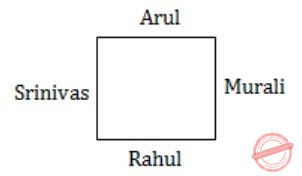Srinivas and Murali and Arul and Rahul are opposite to each other.
They face away from the center of tableEven in this case,
Srinivas and Murali and Arul and Rahul are opposite to each other.
 Question 14

Find the smallest number y such that y × 162 is a prefect cube.

 A 24 B 27 C 32 D 36
Aptitude       Numerical       GATE 2017 [Set-1]       Video-Explanation
Question 14 Explanation:
Prime factorize: 162
⇒ 162 = 2×3×3×3×3 = 33×(2×3)
For (2×3) to be a perfect cube, it should be multiplied by (22×32)
∴ Required number = y = 22×32 = 36
 Question 15

The probability that a k-digit number does NOT contain the digits 0, 5 or 9 is

 A 0.3k B 0.6k C 0.7k D 0.9k
Aptitude       Numerical       GATE 2017 [Set-1]       Video-Explanation
Question 15 Explanation:
Possibilities of doesn't contain 0,5,9 = (7)k
Total no.of possibilities = (10)k
Required probability = (7)k / (10)k = 0.7k
 Question 16

Six people are seated around a circular table. There are atleast two men and two women. There are at least three right-handed persons. Every woman has a left-handed person to her immediate right. None of the women are right-handed. The number of women at the table is

 A 2 B 3 C 4 D Cannot be determined
Aptitude       Numerical       GATE 2017 [Set-1]       Video-Explanation
Question 16 Explanation:
There are atleast two men and two women.
Atleast three right- handed persons.
None of the women are right-handed.
⇒ All the right-handed persons are men. Every woman has a left-handed person to her immediate right.
⇒ For this to happen, there must be three left-handed-persons and one of them should be a male.
As three persons are right-handed and those being men.
The number of women at the table = 2
 Question 17

The expression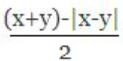is equal to

 A the maximum of x and y B the minimum of x and y C 1 D none of the above
Aptitude       Numerical       GATE 2017 [Set-1]       Video-Explanation
Question 17 Explanation:
As the given expression has modulus, let us consider:
Case-I: x > y
⇒ |x - y| = x - y
Expression ⇒ (x + y) - (x - y) / 2 = y
In this case value of expression is minimum of y.
Case-II: x < y
⇒ |x - y| = -(x - y)
Expression ⇒ (x + y) - (x - y) / 2
⇒ (x + y) - [-(x - y)]/2 ⇒ x
In this case value of expression is minimum of x.
Finally, the value of expression is minimum of x & y.
 Question 18

Arun, Gulab, Neel and Shweta must choose one shirt each from a pile of four shirts coloured red, pink, blue and white respectively. Arun dislikes the colour red and Shweta dislikes the colour white. Gulab and Neel like all the colours. In how many different ways can they choose the shirts so that no one has a shirt with a colour he or she dislikes?

 A 21 B 18 C 16 D 14
Aptitude       Numerical       GATE 2017 [Set-1]       Video-Explanation
Question 18 Explanation:
Total number of ways that each select one shirt without any condition = 4P4 = 4! = 24
Number of ways Arun chooses red or Shweta chooses white
= Number of ways Arun chooses red + Number of ways Shweta chooses white - Number of ways Arun chooses red and Shweta chooses white
= 3P3 + 3P3 - 2P2
= 3! + 3! - 2!
= 10
∴ Required number of ways = 24 - 10 = 14
 Question 19

A contour line joins locations having the same height above the mean sea level. The following is a contour plot of a geographical region. Contour lines are shown at 25 m intervals in this plot. If in a flood, the water level rises to 525 m, which of the villages P, Q, R, S, T get submerged?A P, Q B P, Q, T C R, S, T D Q, R, S
Aptitude       Numerical       GATE 2017 [Set-1]       Video-Explanation
Question 19 Explanation:
The contour lines are shown at 25m interval in this plot.
∴ From the plot,
P=575m
Q=525m
R=475m
S=425m
T=500m
For a village to be submerged, the water level should rise to level greater than the village.
If water level rises to 525m, villages which are at heights below 525m, get submerged.
Here, R, S, T get submerged.
 Question 20

There are five buildings called V, W, X, Y and Z in a row (not necessarily in that order). V is to the West of W, Z is to the East of X and the West of V, W is to the West of Y. Which is the building in the middle?

 A V B W C X D Y
Aptitude       Numerical       GATE 2017 [Set-2]       Video-Explanation
Question 20 Explanation:
V is to west of W = VW .........(i)
Z is to East of X and west of V = XZV ........(ii)
W is to west of Y = WY .........(iii)
From (i) and (ii) ⇒ VWY .........(iv)
From (ii) and (iv) ⇒ XZVWY
→ While building V is in the middle
 Question 21

A test has twenty questions worth 100 marks in total. There are two types of questions. Multiple choice questions are worth 3 marks each and essay questions are worth 11 marks each. How many multiple choice questions does the exam have?

 A 12 B 15 C 18 D 19
Aptitude       Numerical       GATE 2017 [Set-2]       Video-Explanation
Question 21 Explanation:
No. of 3 marks questions = X say
No. of 4 marks questions = Y say
No. of questions X + Y = 20
Total no. of marks = 100
⇒ 3X + 11Y = 100
Option I:
If X=12; Y=8 ⇒ 3(12)+11(8) ⇒ 36+88 ≠ 100
Option II:
If X=15; Y=5 ⇒ 3(15)+11(5) ⇒ 100 = 100
Option III:
If X=18; Y=2 ⇒ 3(18)+11(2) ≠ 100
Option IV:
If X=19; Y=1 ⇒ 3(19)+11(1) ≠ 100
 Question 22

There are 3 red socks, 4 green socks and 3 blue socks. You choose 2 socks. The probability that they are of the same colour is

 A 1⁄5 B 7⁄30 C 1⁄4 D 4⁄15
Aptitude       Numerical       GATE 2017 [Set-2]       Video-Explanation
Question 22 Explanation:
Totally there are 3 red socks, 4 green socks, 3 blue socks. In those we need to select 2 socks
The probability of selecting same colour is
3C2 / 10C2 * 4C2 / 10C2 * 3C2 / 10C2
⇒ 3/45 + 6/45 + 3/45
⇒ 12/45
= 4/15
 Question 23

There are three boxes. One contains apples, another contains oranges and the last one contains both apple and oranges. All three are known to be incorrectly labeled. If you are permitted to open just one box and then pull out and inspect only one fruit, which box would you open to determine the contents of all three boxes?

 A The box labeled ‘Apples’ B The box labeled ‘Apples and Oranges’ C The box labeled ‘Oranges’ D Cannot be determined
Aptitude       Numerical       GATE 2017 [Set-2]       Video-Explanation
Question 23 Explanation:
Correct Answer is option B i.e., the box labeled Apples an Oranges.
If we open a box labeled as apples and oranges that contains either apples (or) oranges:
Case I:
If it contains apples, then the box labeled oranges can contain apples and oranges and box labeled apples can contain oranges.
Case II:
If it contains oranges, then box labeled apples can contains apples and oranges and box labeled oranges can contains apples.
 Question 24

X is a 30 digit number starting with the digit 4 followed by the digit 7. Then the number X3 will have

 A 90 digits B 91 digits C 92 digits D 93 digits
Aptitude       Numerical       GATE 2017 [Set-2]       Video-Explanation
Question 24 Explanation:
X is a 30 digit number starting with digit 4 and followed by the digit 7
X = 4777......... (29 times (7))
X = 4.777........ ×1029
X = 5×1029 (4.777 rounded as 5)
X3 = 125×1087
Total no. of digits = 3+87 [125=3 digit, 1087 = 87 digit]
= 90
 Question 25

The number of roots of ex + 0.5x2 – 2 in the range [-5, 5] is

 A 0 B 1 C 2 D 3
Aptitude       Numerical       GATE 2017 [Set-2]       Video-Explanation
Question 25 Explanation:
ex + 0.5x2 - 2 = 0
ex = -0.5x2 + 2
If we draw a graph for ex and -0.5x2 + 2 in between [-5, 5]Question 26

An air pressure contour line joins locations in a region having the same atmospheric pressure. The following is an air pressure contour plot of a geographical region. Contour lines are shown at 0.05 bar intervals in this plot.If the possibility of a thunderstorm is given by how fast air pressure rises or drops over a region, which of the following regions is most likely to have a thunderstorm?

 A P B Q C R D S
Aptitude       Numerical       GATE 2017 [Set-2]       Video-Explanation
Question 26 Explanation:
Region R can have more air pressure as compared to other regions because it has maximum number of lines crossing the area.
 Question 27

A cube is built using 64 cubic blocks of side one unit. After it is built, one cubic block is removed from every corner of the cube. The resulting surface area of the body (in square units) after the removal is __________.

 A 56 B 64 C 72 D 96
Aptitude       Numerical       GATE 2016 [Set-1]       Video-Explanation
Question 27 Explanation:
64 cubic blocks are used to make the cube
⇒ Side of cube = 4
Surface area of cube = 6s2 = 6 × 42 = 96
Each corner block is associated with three faces of cube.
When they are removed, three new faces are exposed. Thus there appears no net change in the exposed surface area.
Hence, Surface area = 96
 Question 28

A shaving set company sells 4 different types of razors, Elegance, Smooth, Soft and Executive. Elegance sells at Rs. 48, Smooth at Rs. 63, Soft at Rs. 78 and Executive at Rs. 173 per piece. The table below shows the numbers of each razor sold in each quarter of a year.Which product contributes the greatest fraction to the revenue of the company in that year?

 A Elegance B Executive C Smooth D Soft
Aptitude       Numerical       GATE 2016 [Set-1]       Video-Explanation
Question 28 Explanation:
Revenue from Elegance = (27300 + 25222 + 28976 + 21012) × 48 = Rs. 4920480
Revenue from Smooth = (20009 + 19392 + 22429 + 18229) × 63 = Rs. 5043717
Revenue from Soft = (17602 + 18445 + 19544 + 16595) × 78 = Rs.5630508
Revenue from Executive = (9999 + 8942 + 10234 + 10109) × 173 = Rs. 6796132
Clearly, Executive contributes the greatest fraction to the revenue of the company as the revenue from it is the highest.
 Question 29

Consider the following statements relating to the level of poker play of four players P, Q, R and S.

I. P always beats Q
II. R always beats S
III. S loses to P only sometimes
IV. R always loses to Q

Which of the following can be logically inferred from the above statements?

(i) P is likely to beat all the three other players
(ii) S is the absolute worst player in the set
 A (i) only B (ii) only C (i) and (ii) D neither (i) nor (ii)
Aptitude       numerical       GATE 2016 [Set-1]       Video-Explanation
Question 29 Explanation:
From statements I, II & IV, we can say that
All three can beat S.
But from statement III,
S loses to P only sometimes.
(ii) Cannot be inferred.
And in poker, the transitive law does not apply. This can be seen from statement III.
As S loses to P only sometimes which states that wins against P most of the time.
So, (i) cannot be logically inferred.
 Question 30

If f(x) = 2x7 + 3x - 5, which of the following is a factor of f(x)?

 A (x 3 +8) B (x-1) C (2x-5) D (x+1)
Aptitude       Numerical       GATE 2016 [Set-1]       Video-Explanation
Question 30 Explanation:
f(x) = 2x7 + 3x - 5
For (x - a) to be a factor of f(x)
f(a) = 0
From options,
Only a=1, satisfies the above equation
∴ (x - 1) is a factor of f(x).
 Question 31

In a process, the number of cycles to failure decreases exponentially with an increase in load. At a load of 80 units, it takes 100 cycles for failure. When the load is halved, it takes 10000 cycles for failure. The load for which the failure will happen in 5000 cycles is ________.

 A 40 B 46.02 C 60.01 D 92.02
Aptitude       numerical       GATE 2016 [Set-1]       Video-Explanation
Question 31 Explanation:
In a process, the number of cycles to failure decrease exponentially with an increase in load.
General exponential function = a⋅e-bx
i.e., No. of cycles to failure = a⋅e-bx
At a load of 80 units, it takes 100 cycles to failure.
So, no. of cycles to failure = 100
i.e., 100 = a⋅e - b(80) --------(1)
When the load is halved, it takes 10000 cycles to failure.
No. of cycles to failure = 10,000
i.e., 10,000 = a⋅e - b(40) ---------(2)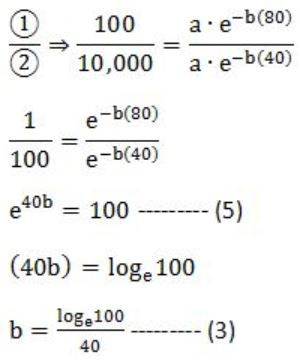No. of cycles to failure = 5,000
i.e., 5000 = a⋅e - bx
Multiply with 2 on both sides,
10,000 = 2⋅a⋅e - bx ------- (4)Question 32

Pick the odd one from the following options.

 A CADBE B JHKIL C XVYWZ D ONPMQ
Aptitude       Numerical       GATE 2016 [Set-2]       Video-Explanation
Question 32 Explanation:
In all the given options except D, the 1st, 3rd, 5th alphabets are consecutive (increasing order) and 2nd and 4th are consecutive (increasing order).
But in D, 2nd and 4th are in decreasing order.
 Question 33

In a quadratic function, the value of the product of the roots (α, β) is 4. Find the value of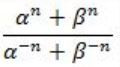A n4 B 4n C 22n-1 D 4n-1
Aptitude       Numerical       GATE 2016 [Set-2]       Video-Explanation
Question 33 Explanation:
Given,
Product of roots (α, β) = 4
⇒ αβ = 4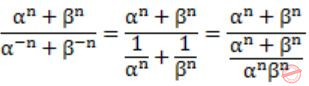(αβ)n = 4n
 Question 34

Among 150 faculty members in an institute, 55 are connected with each other through Facebook® and 85 are connected through WhatsApp®. 30 faculty members do not have Facebook® or WhatsApp® accounts. The number of faculty members connected only through Facebook® accounts is ______________.

 A 35 B 45 C 65 D 90
Aptitude       Numerical       GATE 2016 [Set-2]       Video-Explanation
Question 34 Explanation:
Total number of faculty = 150
Number of faculty members connected through Facebook = 55
Number of faculty members connected through Whatsapp = 85
Number of faculty members with Facebook (or) Whatsapp accounts = 30
Number of faculty members with either Facebook (or) Whatsapp accounts = 150 - 30 = 120
Number of faculty members with both Facebook and Whatsapp accounts = 85 + 55 - 120 = 20
Number of faculty members with only Facebook accounts = 55 - 20 = 35
 Question 35

In a 2 × 4 rectangle grid shown below, each cell is a rectangle. How many rectangles can be observed in the grid?A 21 B 27 C 30 D 36
Aptitude       Numerical       GATE 2016 [Set-2]       Video-Explanation
Question 35 Explanation:
Number of rectangles in a grid with 'm' horizontal and 'n' vertical lines = 5C2 × 3C2 = 10 × 3 = 30
 Question 36Choose the correct expression for f(x) given in the graph.

 A f(x) = 1 - |x - 1| B f(x) = 1 + |x - 1| C f(x) = 2 - |x - 1| D f(x) = 2 + |x - 1|
Aptitude       Numerical       GATE 2016 [Set-2]       Video-Explanation
Question 36 Explanation:
From the graph,
x = -1 ⇒ f(x) = 0
x = 0 ⇒ f(x) = 1
We have check, which option satisfies both the conditions.
Only option (C) satisfies both of them.
 Question 37

Given set A = {2, 3, 4, 5} and Set B = {11, 12, 13, 14, 15}, two numbers are randomly selected, one from each set. What is probability that the sum of the two numbers equals 16?

 A 0.2 B 0.25 C 0.3 D 0.33
Aptitude       Numerical       GATE 2015 [Set-1]
Question 37 Explanation:
Favourable outcomes = {2,14}, {3,13}, {4,12}, {5,11} = 4
Total outcomes = 4C1 × 5C1 = 20
Probability = Favourable outcomes/ Total outcomes = 4/20 = 0.20
 Question 38

Based on the given statements, select the most appropriate option to solve the given question.

If two floors in a certain building are 9 feet apart, how many steps are there in a set of stairs that extends from the first floor to the second floor of the building?

Statements:
(I) Each step is 3/4 foot high.
(II) Each step is 1 foot wide.
 A Statement I alone is sufficient, but statement II alone is not sufficient. B Statement II alone is sufficient, but statement I alone is not sufficient. C Both statements together are sufficient, but neither statement alone is sufficient. D Statement I and II together are not sufficient.
Aptitude       Numerical       GATE 2015 [Set-1]
Question 38 Explanation:
When you climbing, only height matters not the width.
Statement I:
Each step is 3/4 foot high.
No. of steps = 9/(3/4) = 12 steps
Statement I alone is sufficient.
Statement II:
Each step is 1 foot wide.
Statement II alone is not sufficient.
 Question 39

The pie chart below has the breakup of the number of students from different departments in an engineering college for the year 2012. The proportion of male to female students in each department is 5:4. There are 40 males in Electrical Engineering. What is the difference between numbers of female students in the Civil department and the female students in the Mechanical department?A 32 B 33 C 34 D 35
Aptitude       Numerical       GATE 2015 [Set-1]
Question 39 Explanation:
Male to female students in each department = 5 : 4
There are 40 males in Electrical Engineering.
⇒ Number of females in Electrical Engineering = 4/5 × 40 = 32
Total number of students in Electrical Engineering = 40+32 = 72
This constitutes 20% of the strength of college.
Number of students in Civil department = 30/100 × 72 = 108
Number of female students in Civil department = 4/(4+5) × 108 = 48
Number of students in Mechanical department = 10/20 × 72 = 36
Number of female students in Mechanical department = 4/(4+5) × 36 = 16
Required Difference = 48 - 16 = 32
 Question 40

The probabilities that a student passes in Mathematics, Physics and Chemistry are m, p and c respectively. Of these subjects, the student has 75% chance of passing in at least one, a 50% chance of passing in at least two and a 40% chance of passing in exactly two. Following relations are drawn in m, p, c:

(I) p + m + c = 27/20
(II) p + m + c = 13/20
(III) (p) × (m) × (c) = 1/10
 A Only relation I is true B Only relation II is true C Relations II and III are true. D Relations I and III are true.
Aptitude       Numerical       GATE 2015 [Set-1]
Question 40 Explanation:
75% of students have a chance of passing atleast one subject
= 1 - (1 - m) (1 - p) (1 - c) = 0.75 ----(i)
50% of students have a chance pass in atleast two
(1 - m)pc + (1 - p)mc + (1 - c) mp + mpc = 0.5 ----(ii)
40% of students have a chance of passing exactly two
(1 - m)pc + (1 - p)mc + (1 - c)mp = 0.4 ----(iii)
From equation (ii) and (iii) we can get
mpc = 0.1
⇒ m*p*c = 1/10
Statement (III) is correct.
→ Simplify eq (i), we get
⇒ p+c+m - (mp+mc+pc) + mpc = 0.75
⇒ p+c+m - (mp+mc+pc) = 0.65 ----(iv) → Simplify equation (iii), we get
⇒ pc + mc + mp - 3mpc = 0.4
From (iv) and (v)
p + c + m - 0.7 = 0.65
⇒ p + c + m = 1.35 = 27/20
Statement (I) is correct.
 Question 41

The number of students in a class who have answered correctly, wrongly, or not attempted each question in an exam, are listed in the table below. The marks for each question are also listed. There is no negative or partial marking.What is the average of the marks obtained by the class in the examination?

 A 2.29 B 2.97 C 6.795 D 8.795
Aptitude       Numerical       GATE 2015 [Set-1]
Question 41 Explanation:
Total number of students = 44 (Answered- correctly + Answered-wrongly + Not-attempted)
Total marks obtained = 21 × 2 + 15 × 3 + 11 × 1 + 23 × 2 + 31 × 5 = 299
Average marks = 299/44 = 6.795
 Question 42

Based on the given statements, select the most appropriate option to solve the given question, What will be the total weight of 10 poles each of same weight?

Statements:
(I) One fourth of the weight of a pole is 5 kg
(II) The total weight of these poles is 160 kg more than the total weight of two poles.
 A Statement I alone is not sufficient B Statement II alone is not sufficient C Statement II alone is not sufficient D Both statements I and II together are not sufficient.
Aptitude       Numerical       GATE 2015 [Set-2]
Question 42 Explanation:
Statement-I:
One fourth of the weight of a pole is 5Kg. ⇒ Weight of pole is 4×5 = 20Kg
Weight of 10 poles each of same weight = 10×20 = 200 Kg
∴Statement I alone is sufficient.
Statement-II:
Let, Weight of each pole = W Kg
Given,
10W = 2W + 160
⇒ 8W = 160
W = 20Kg
∴ Weight of each pole = 20 Kg
∴ Weight of 10 poles = 10×20 Kg = 200 Kg
∴ Statement II alone is sufficient.
Either I or II alone is sufficient.
 Question 43

Consider a function f(x) = 1 - |x| on -1 ≤ x ≤ 1. The value of x at which the function attains a maximum and the maximum value of the function are

 A 0, -1 B -1, 0 C 0, 1 D -1, 2
Aptitude       Numerical       GATE 2015 [Set-2]
Question 43 Explanation:
f(x) = 1 - |x| on -1 ≤ x ≤ 1
In the given function, it is given as
-|x| ⇒ To obtain the maximum of this function we have to minimize the value -|x| and the minimum value is 0.
∴ Maximum value of f(x) is at f(0) and i.e., f(x) = 1
Maximum value is 1 at x=0.
 Question 44

In a triangle PQR, PS is the angle bisector of ∠QPR and ∠QPS = 60º. What is the length of PS?A (q+r)/qr B qr/(q+r) C √(q2 + r2) D (q+r)2 /qr
Aptitude       Numerical       GATE 2015 [Set-2]
Question 44 Explanation:
Given,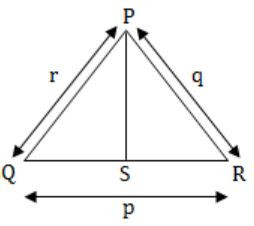∠QPS = 60º
and PS is angle bisector of ∠QPR
⇒ ∠QPS = ∠SPR = 60º
and ∠QPR = 120º
Let, PS = s
Area of ΔPQR = Area of ΔPQS + Area of ΔSPR
1/2 qr Sin∠QPR = 1/2 rs Sin∠QPS + 1/2 sq∠SPR
1/2 qr Sin 120º = 1/2 rs Sin 60º + 1/2 sq 60º
1/2 qr Sin × √3/2 = 1/2 rs × √3/2 + 1/2 × √3/2
qr = rs + sq
∴ s = qr/q+r
PS = qr/q+r
 Question 45

If the list of letters, P, R, S, T, U is an arithmetic sequence, which of the following are also in arithmetic sequence?

I. 2P, 2R, 2S, 2T, 2U
II. P-3, R-3, S-3, T-3, U-3
III. P2, R2, S2, T2, U2
 A I only B I and II C II and III D I and III
Aptitude       Numerical       GATE 2015 [Set-2]
Question 45 Explanation:
If any set of numbers are in a arithmetic sequence and if a common number if added (or) subtracted from each of these numbers, the new set will also be in arithmetic sequence.
Hence, II is an arithmetic sequence.
If the set of numbers is multiplied by the common number, even then the new set will also be in arithmetic sequence.
Hence, I is an arithmetic sequence.
 Question 46

If p, q, r, s are distinct integers such that:

f(p,q,r,s) = max(p,q,r,s)
g(p,q,r,s) = min(p,q,r,s)
h(p,q,r,s) = remainder of (p×q)(r×s) if (p×q)>(r×s) OR remainder of (r×s)(p×q) if (r×s)>(p×q)

Also a function fgh(p,q,r,s) = f(p,q,r,s) × g(p,q,r,s) ×h(p,q,r,s)

Also the same operations are valid with two variable functions of the form f(p,q).

What is the value of fg(h(2,5,7,3),(4,6,8)?

 A 8 B 9 C 7 D 6
Aptitude       Numerical       GATE 2015 [Set-2]
Question 46 Explanation:
h(2,5,7,3) = remainder of (7×3)/(2×5)
= remainder of 21/10
= 1
fg(1,4,6,8) = f(1,4,6,8) × g(1,4,6,8)
= max(1,4,6,8) × min(1,4,6,8)
= 8 × 1
= 8
 Question 47

Four branches of a company are located at M, N, O and P. M is north of N at a distance of 4km; P is south of O at a distance of 2 km; N is southeast of O by 1 km. What is the distance between M and P in km?

 A 5.34 B 6.74 C 28.5 D 45.49
Aptitude       Numerical       GATE 2015 [Set-2]
Question 47 Explanation: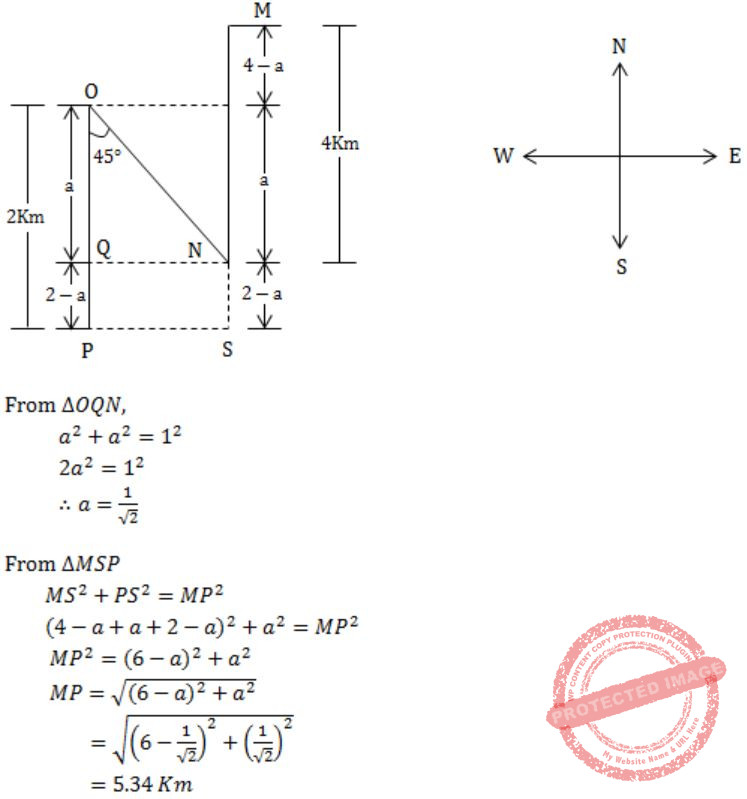Question 48

An unordered list contains n distinct elements. The number of comparisons to find an element in this list that is neither maximum nor minimum is

 A Θ(nlog n) B Θ(n) C Θ(log n) D Θ(1)
Aptitude       Numerical       GATE 2015 [Set-2]
Question 48 Explanation:
Consider first three element of the list, atleast one of them will be neither minimum nor maximum. ∴ Θ(1)
 Question 49

A function f(x) is linear and has a value of 29 at x = -2 and 39 at x = 3. Find its value at x = 5.

 A 59 B 45 C 43 D 35
Aptitude       Numerical       GATE 2015 [Set-3]
Question 49 Explanation:
f(x) = 2x + 33
 Question 50

If ROAD is written as URDG, then SWAN should be written as:

 A VXDQ B VZDQ C VZDP D UXDQ
Aptitude       Numerical       GATE 2015 [Set-3]
Question 50 Explanation:
R+3 = U, O+3 = R, A+3 = D, D+3 = G;
S+3 = V, W+3 = Z, A+3 = D, N+3 = Q.
 Question 51

The exports and imports (in crores of Rs.) of a country from the year 2000 to 2007 are given in the following bar chart. In which year is the combined percentage increase in imports and exports the highest?A 2006 B 2007 C 2008 D 2009
Aptitude       Numerical       GATE 2015 [Set-3]
Question 51 Explanation:
Increase in exports in 2006 = 100 - 70/70 = 42.8%
Increase in imports in 2006 = 120 - 90/90 = 33.3% which is more than any other year.
There are 51 questions to complete.

Register Now